# Sine - math problems

#### Number of problems found: 167

• Sin cos tan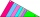In triangle ABC, right-angled at B. Sides/AB/=7cm, /BC/=5cm, /AC/=8.6cm. Find to two decimal places. A. Sine C B. Cosine C C. Tangent C.
• Angles by cosine lawCalculate the size of the angles of the triangle ABC, if it is given by: a = 3 cm; b = 5 cm; c = 7 cm (use the sine and cosine theorem).
• Right triangle trigonometricsCalculate the size of the remaining sides and angles of a right triangle ABC if it is given: b = 10 cm; c = 20 cm; angle alpha = 60° and the angle beta = 30° (use the Pythagorean theorem and functions sine, cosine, tangent, cotangent)
• The cone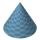The lateral surface area of the cone is 4 cm2, the area of the base of the cone is 2 cm2. Determine the angle in degrees (deviation) of the cone sine and the cone base plane. (Cone side is the segment joining the vertex cone with any point of the base c
• TrigonometryIs true equality? ?
• Q-Exam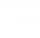If tg α = 9.6, Calculating sin α, cos α, cotg α .
• Height 2Calculate the height of the equilateral triangle with side 27.
• CotangentIf the angle α is acute, and cotan α = 1/3. Determine the value of sin α, cos α, tg α.
• One side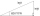One side is 36 long with a 15° incline. What is the height at the end of that side?
• SinesIn ▵ ABC, if sin(α)=0.5 and sin(β)=0.6 calculate sin(γ)
• Sinus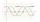Determine the smallest integer p for which the equation 4 sin x = p has no solution.
• Trigonometric functionsIn the right triangle is: ? Find the value of s and c: ? ?
• PentagonCalculate the area of a regular pentagon, which diagonal is u=16.
• RightDetermine angles of the right triangle with the hypotenuse c and legs a, b, if: ?
• ABCDAC= 40cm , angle DAB=38 , angle DCB=58 , angle DBC=90 , DB is perpendicular on AC , find BD and ADThe ladder has a length of 3 m and is leaning against the wall, and its inclination to the wall is 45°. How high does it reach?
• TrigonometryIf you know that cos(γ) = sin (806°), what is the angle γ?
• Right triangleA right triangle ABC is given, c is a hypotenuse. Find the length of the sides a, b, the angle beta if c = 5 and angle alfa = A = 35 degrees.What is the slope of a ladder 6.2 m long and 5.12 m in height.
• RailwayRailway line had on 5.8 km segment climb 9 permille. How many meters track ascent?

Do you have an interesting mathematical word problem that you can't solve it? Submit a math problem, and we can try to solve it.

We will send a solution to your e-mail address. Solved examples are also published here. Please enter the e-mail correctly and check whether you don't have a full mailbox.

Please do not submit problems from current active competitions such as Mathematical Olympiad, correspondence seminars etc...

Most natural application of trigonometry and trigonometric functions is a calculation of the triangles. Common and less common calculations of different types of triangles offers our triangle calculator. Word trigonometry comes from Greek and literally means triangle calculation.